什么是计数排序？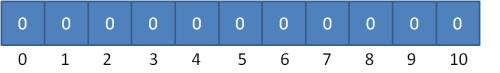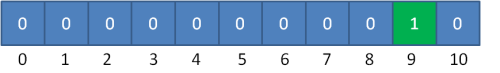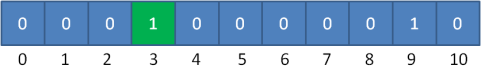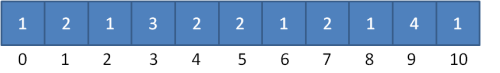0, 1, 1, 2, 3, 3, 3, 4, 4, 5, 5, 6, 7, 7, 8, 9, 9, 9, 9, 10

public static int[] countSort(int[] array) {
//1.得到数列的最大值
int max = array;
for (int i = 1; i < array.length; i++) {
if (array[i] > max)
max = array[i];
}
//2.根据数列的最大值确定统计数组的长度
int[] coutArray = new int[max + 1];
//3.遍历数列，填充统计数组
for(int i = 0; i < array.length; i++)
coutArray[array[i]]++;

//4.遍历统计数组，输出结果
int index = 0;
int[] sortedArray = new int[array.length];
for (int i = 0; i < coutArray.length; i++) {
for (int j = 0; j < coutArray[i]; j++) {
sortedArray[index++] = i;
}
}

return sortedArray;
}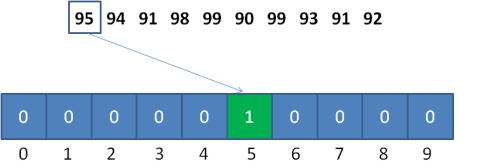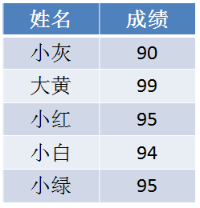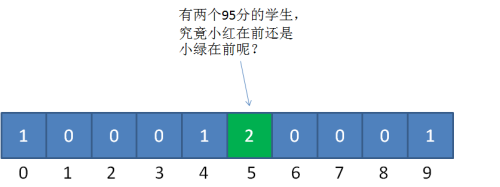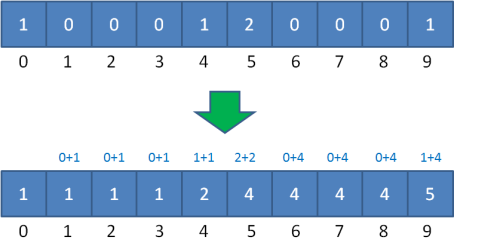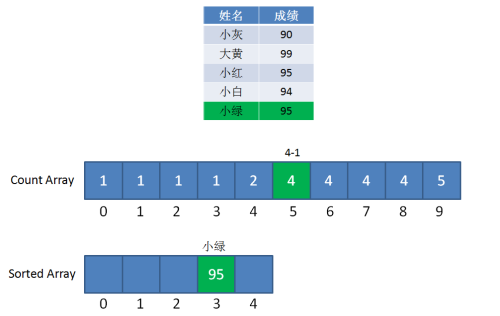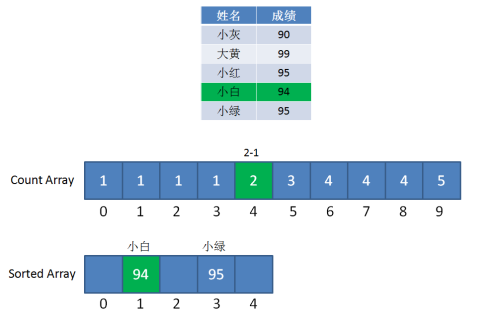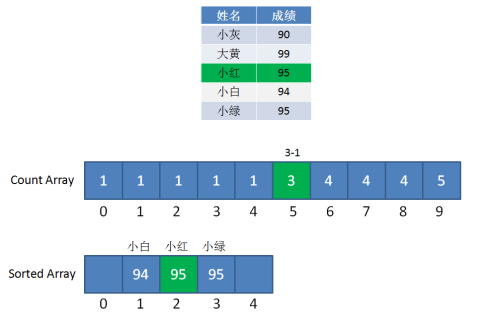public static int[] countSort(int[] array) {
//1.得到数列的最大值与最小值，并算出差值d
int max = array;
int min = array;
for (int i = 1; i < array.length; i++) {
if (array[i] > max) {
max = array[i];
}
if(array[i] < min) {
min = array[i];
}
}
int d = max - min;
//2.创建统计数组并计算统计对应元素个数
int[] countArray = new int[d + 1];
for (int i = 0; i < array.length; i++) {
countArray[array[i] - min]++;
}
//3.统计数组变形，后面的元素等于前面的元素之和
int sum = 0;
for (int i = 0; i < countArray.length; i++) {
sum += countArray[i];
countArray[i] = sum;
}
//4.倒序遍历原始数组，从统计数组找到正确位置，输出到结果数组
int[] sortedArray = new int[array.length];
for (int i = array.length - 1; i > 0; i--) {
sortedArray[countArray[array[i] - min] - 1] = array[i];
countArray[array[i] - min]--;
}
return sortedArray;
}

1.当数列最大最小值差距过大时，并不适用于计数排序

2.当数列元素不是整数时，并不适用于计数排序

posted @ 2019-03-26 23:50 murphy_gb 阅读(...) 评论(...) 编辑 收藏